# The impacts of heat generation/absorption and partial slip on boundary layer flow and heat transfer of a nanofluid comprising of self-impelled motile microorganisms passing a stretching sheet

The impacts of heat generation/absorption and partial slip on boundary layer flow and heat transfer of a nanofluid comprising of self-impelled motile microorganisms passing a stretching sheet

Mohsen IzadiSeyed Abdollah Mansouri MehryanAli J. ChamkhaGiulio Lorenzini

Mechanical Engineering Department, Faculty of Engineering, Lorestan University, Khorramabad, P.O. Box 68151-44316, Iran

Young Researchers and Elite Club, Yasooj Branch, Islamic Azad University, Yasooj, Iran

Mechanical Engineering Department, Prince Sultan Endowment for Energy and Environment, Prince Mohammad Bin Fahd University, Al-Khobar 31952, Saudi Arabia

RAK Research and Innovation Center, American University of Ras Al Khaimah, United Arab Emirates

Department of Engineering and Architecture, University of Parma, Parco Area delle Scienze, 181/A, Parma 43124, Italy

Corresponding Author Email:
Page:
10-20
|
DOI:
https://doi.org/10.18280/mmep.060102
15 September 2018
|
Accepted:
16 January 2019
|
Published:
31 March 2019
| Citation

OPEN ACCESS

Abstract:

The present paper has conducted the numerical analysis of a nanofluid consisting of microorganisms on the isothermal linear stretching sheet. The problem has been modeled under the influences of partial slip and heat absorption/generation at the sheet surface. The governing equations are converted to ordinary differential equations through a series of similarity transformations. Using a finite difference method, the resulting equations are discretized, and also they are linearized by applying the linearization technique of Newton. Results of temperature, velocity, concentration of nanoparticles, motile microorganisms’ density and also coefficient of reduced skin friction, reduced Nu, reduced Shr, and reduced density numbers of the microorganisms are graphically demonstrated and a detailed description is given. The microorganisms dimensionless density increases as δ increase, while decreases with increasing the values of Pe, Lb, Gr and Ω. Also, boundary layer thickness for motile microorganisms declines by increasing Lb and Ω. Finally, when the rates of heat generation(λ>0) and absorbsion (λ<0) increase, the microorganisms density increases and decreases, respectively.

Keywords:

nanofluid, stretching sheet, motile gyrotactic microorganisms, heat generation/absorption, partial slip

1. Introduction

In recent decade, a relatively new topic called nanofluids that are very important for many practical applications in the modern industry has attracted numerous researches [1–7]. The evaluation of flow and heat transfer on the stretching surfaces has attracted numerous researchers and experts at world-wide. different industrial application of such process are optical fibers, paper cooling, rubber sheet and plastic stretching, cooling of metallic sheets, crystal growing, vulcanite sheets and polymer extrusion [8-10]. The rate of stretching and cooling, in the process, has incontrovertible effects on the quality of the resulting product.

Analysis of boundary layer flow passing a plate with an invariable velocity started by Sakiadis . Later, numerous papers studied the flow of boundary layer over the stretching surfaces by taking into account various phenomena, including blowing or suction, porosity and magnetic field and various type of fluids, for example: polar, Newtonian, and non-Newtonian. Le Erickson et al.  continued Sakiadis’s work. They examined the influence of heat exchange, suction and blowing on flow. First, Crane  checked out the boundary layer flow passing on a moving plate that over it; the distance from the slit edge was proportional to velocity. Char  conducted the investigation of fluid’s flow and heat exchange passing on a permeable stretching surface.

Assessment of flow and heat transfer in a composed boundary layer with considering the passing of nanofluids over a stretching surface contains the broad scope of recent investigations. The most significant subject in the boundary layer flow is the characteristic of heat exchange namely the surface’s heat transfer rate. Because the final product’s quality is proportional to the rate of heat transfer. However, traditional heat-transfer fluids, for example engine oil, and water, have a rather low thermal conductivity. in order to solve this problem, the use of high thermal conductive solid nanoparticles dispersed in the base-fluid, has been selected by many researches. The presence of these nanoparticles within the resulting fluid is likely to improve the thermal conductivity. Kuznetsov and Nield  conducted an analytical investigation of the free convective heat exchange of a nanofluid passing a vertical sheet. In simulation, thermophoresis influences and Brownian motion were taken into account. Khan and Pop  assessed the boundary layer flow of a nanofluid passing an isothermal stretching surface. In another study, Khan et al.  investigated the flow and heat transfer of ferrofluid on a flat surface considering the influence of the slip velocity. Makinde and Aziz  performed a numeric investigation on nanofluid flow passing a stretching sheet. Ibrahim and Shankar  evaluated the flow and heat exchange in a boundary layer of a magnetic nanofluid along a continuous stretching sheet. Ferdows et al.  analyzed the nanofluid flow passing stretching surface under the influences of radiation and magnetic field. Vajravelu et al.  checked out the flow of Cu-water and Ag-water nanofluid passing a continuous stretching solid surface. Mabood and Das  conducted investigation of the magneto-hydrodynamic flow and also heat exchange of a melting nanofluid passing a stretching surface by addressing heat radiation and second order slip model. Entropy generation of a nanofluid flow on a nonlinear stretching inclined surface in a permeable media under the variable magnetic field, viscous dissipation, and solar radiation is numerically analyzed by Dehsara et al. . Some other relevant researches have discussed various sights of nanofluids passing a stretching sheet [24–30].

The bio-convection macroscopically means the convective motion of a fluid that is subjected to the generated gradient of density through plural swimming and also movement of microorganisms [31–34]. The self-impelled motile microorganisms boost the density of host fluid in a specific orientation so that results in the bio-convection flow. In general, it can be said that the microorganisms swimming is a reaction to the external force field, for instance, gravity or biochemical stimulus such as oxygen concentration gradient . Nanoparticles are not self-impelled, quite contradictory to motile microorganisms, and their movement is the result of the effects of Brownian movement and thermophoresis in nanofluid. Kuznetsov [35–37] was among the first people who proposed the matter of bio-convection through nanofluids. Kuznetsov  confirmed that using nanofluids containing gyrotactic microorganisms increases the mixing of fluid as a result of fluid macroscopic motion created by self-impelled motile microorganisms and prevents the nanoparticles accumulation in nanofluid. Aziz et al.  checked out the flow of boundary layer in a permeable media saturated by nanofluid consisting of motile microorganisms. hydromagnetic flow of combined Nanofluids-microorganisms passing vertical permeable surface is studied by Mutuku and Makinde . Khan et al.  evaluated the natural convection of non-Newtonian nanofluid including microorganisms in a porous media. Most recently, considering existence of a magnetic field behavior of a nanofluid consists of microorganisms over stretching surface is numerically examined by our group .

The no-slip condition of viscous fluids means the fluid andsolid boundaries have the same velocity. The no-slip boundary condition is in fact the main principle of the Navier–Stokes theorem. However, there are exceptions that the condition is not true. Specifically, the no-slip boundary condition is not a proper one for a lot of non-Newtonian fluids and nanofluids [42–44]. In some usages such as Teflon coating, artificial heart valves polishing and the internal cavities withstanding adhesion, it is not possible to hold the no-slip boundary condition inflict on the tangential velocity. In addition, due to roughness or permeability of some surfaces, equivalent slip happens . Moreover, for rarefied gases, there is a hydrodynamic boundary slip regime when the pressure of the flow is extremely low . An extensive number of researches were accomplished to include slip condition. Navier  discussed the slip boundary condition of fluid in which the velocity of slip linearly depends on shear stress. Fang et al.  perused the MHD viscous flow by considering the slip of fluid passing from the stretching sheet. Also, boundary layer and heat exchange along a stretching surface with the second-order slip boundary condition is analyzed by some researchers [49–54] employed a partially slip boundary condition in order to investigate the fluid flow passing stretching surface. The boundary layer flow resulting from the movement of fluid over a stretching surface with thermal and hydrodynamics partial slip is performed by Aziz . Noghrehabadi  conducted the study on the entropy generation of a nanofluid passing a stretching surface under the effects of heat generation/absorption. Most recently, some important researches have been done in this field [57–59].

The present paper deals with the effect of the heat generation/absorption presence and partial slip on flow and thermal indexes through boundary layer of combined nanofluid-microorganisms past a stretching surface. This work has, for the first time, examined the transport phenomenon in a nanofluid including gyrotactic microorganisms in the presence of partial slip and the process of convective cooling. The Brownian movement and thermophoresis of nanoparticles are taken into account. To prove the validity, numeric solutions are provided and a comparison with literature Noghrehabadi  is included as well. Employing a finite difference method, a novel collection of equations is analyzed, and by Newton linearization method, they are linearized. Afterwards, results for different physical variables are explained in graphical form and characterized with details.

2. Defining the Problem and Governing Equations

The steady state, laminar and 2D boundary layer of a nanofluid consisting of motile gyrotactic microorganisms over a stretching surface under heat generation/absorption and partial slip was numerically investigated. The suspension of the nanoparticles is stable so that no nanoparticle agglomeration and accumulation occur. Moreover, the assumption is that the nanoparticles do not affect the direction and velocity of swimming microorganisms. The assumption is reasonable when the nanofluid is dilute. If the volume fraction of nanoparticles be under 1%, it is considered as dilute suspension . Bio-convection only arises when there is a dilute nanofluid, because a large volume fraction of nanoparticles increases the fluid viscosity and hence the flow of bio-convection becomes suppressed.

## 1.jpgFigure 1. Physical model of the problem and Cartesian coordinate 

Figure 1 illustrates the physical model of present problem. Temperature (Tw), nanoparticles volume fraction (Cw) and microorganisms concentration (nw) are constant over the stretching surface. The surface velocity is linearly taken as u=ax. The ambient temperature, nanoparticles volume fraction and microorganisms concentration are respectively T∞, C∞ and n∞. Also, it is considered the Oberbeck-Boussinesq approximation is acceptable.

With respect to the above-mentioned considerations, the governing equations  can be represented as:

Conservation of mass:

$\frac{\partial u}{\partial x}+\frac{\partial v}{\partial y}=0$ (1)

Conservation of momentum:

\begin{align} & {{\rho }_{f}}(u\frac{\partial u}{\partial x}+v\frac{\partial u}{\partial y})=-\frac{\partial P}{\partial x}+\mu {{\nabla }^{2}}u+\left( 1-{{C}_{\infty }} \right){{\rho }_{f}}g\beta \left( T-{{T}_{\infty }} \right) \\ & -g\left( {{\rho }_{p}}-{{\rho }_{f}} \right)\left( C-{{C}_{\infty }} \right)-g\gamma \left( {{\rho }_{m}}-{{\rho }_{f}} \right)\left( n-{{n}_{\infty }} \right) \\\end{align} (2)

$\frac{\partial P}{\partial y}=0$ (3)

Conservation of energy:

$u\frac{\partial T}{\partial x}+v\frac{\partial T}{\partial y}=\alpha {{\nabla }^{2}}T+\tau \left( {{D}_{B}}\frac{\partial C}{\partial y}\frac{\partial T}{\partial y}+{{D}_{B}}\frac{\partial C}{\partial x}\frac{\partial T}{\partial x}+\frac{{{D}_{T}}}{{{T}_{\infty }}}{{((\frac{\partial T}{\partial x})+(\frac{\partial T}{\partial y}))}^{2}} \right)+\frac{\mu \alpha }{k}{{(\frac{\partial u}{\partial y})}^{2}}+\frac{Q}{{{\left( \rho {{c}_{p}} \right)}_{f}}}\left( T-{{T}_{\infty }} \right)$ (4)

Concentration of nanoparticles:

$u\frac{\partial C}{\partial x}+v\frac{\partial C}{\partial y}=\alpha {{\nabla }^{2}}C+\frac{{{D}_{T}}}{{{T}_{\infty }}}{{\nabla }^{2}}T$ (5)

Density of motile microorganisms:

\begin{align} & u\frac{\partial n}{\partial x}+v\frac{\partial n}{\partial y}+\frac{b{{W}_{c}}}{\left( {{C}_{w}}-{{C}_{\infty }} \right)}\left[ \frac{\partial }{\partial y}\left( n\frac{\partial C}{\partial y} \right)+\frac{\partial }{\partial x}\left( n\frac{\partial C}{\partial x} \right) \right] \\ & ={{D}_{m}}\left( {{\nabla }^{2}}n+2\frac{{{\partial }^{2}}n}{\partial x\partial y} \right) \\\end{align} (6)

the pressure term deduced from momentum equations. By integrating of the equation in y-direction and using boundary condition at infinity , the simplified form of momentum equation can rewrite as follow:

\begin{align} & {{\rho }_{f}}\left( u\frac{\partial u}{\partial x}+v\frac{\partial u}{\partial y} \right)=\mu {{\nabla }^{2}}u+\left( 1-{{C}_{\infty }} \right){{\rho }_{f}}g\beta \left( T-{{T}_{\infty }} \right) \\ & -g\left( {{\rho }_{p}}-{{\rho }_{f}} \right)\left( C-{{C}_{\infty }} \right)-g\gamma \left( {{\rho }_{m}}-{{\rho }_{f}} \right)\left( n-{{n}_{\infty }} \right) \\\end{align} (7)

The related boundary conditions can be express as following form:

\begin{align} & u={{U}_{w}}\left( x \right)+L\frac{\partial u}{\partial y},{{~}^{{}}}v=0,{{~}^{{}}}T={{T}_{w}}{{,}^{{}}}~C={{C}_{w}},~ \\ & ^{{}}n={{n}_{w}}{{~}^{{}}}at{{~}^{{}}}x=0, \\ & u\to 0,{{~}^{{}}}v\to 0{{,}^{{}}}~T\to {{T}_{\infty }},{{~}^{{}}}n\to {{n}_{\infty }}^{{}}~at{{~}^{{}}}x\to \infty \\\end{align} (8)

In the above equations, u and v are the components of nanofluid velocity in x and y directions, respectively. ρp, ρf and ρm are the density of nanoparticles, the host-fluid density and the microorganisms density, respectively, T and P are temperature and pressure, μ, α and β are the dynamic viscosity, thermal diffusion and volumetric expansion coefficient of base fluid, respectively, g is the gravity acceleration, C and n are the concentration of nanoparticles and microorganisms density, respectively. Also, γ is the mean volume of a microorganism, τ is the ratio of nanoparticles to the base fluid effective heat capacity, DT, DB and Dm are the thermophoresis, Brownian and microorganisms diffusion coefficients, respectively, Q is heat generation/absorption, L is the partial slip coefficient and finally, bWC, b and WC are the constant, chemotaxis constant and maximum cell swimming speed, respectively.

\begin{align} & \eta ={{\left( \frac{a}{\nu } \right)}^{0.5}}y\text{,}\psi ={{\left( a\nu \right)}^{0.5}}xf\left( \eta \right),\theta \left( \eta \right)=~\frac{T-{{T}_{\infty }}}{{{T}_{w}}-{{T}_{\infty }}},\varphi \left( \eta \right)=~\frac{C-{{C}_{\infty }}}{{{C}_{w}}-{{C}_{\infty }}}, \\ & \chi \left( \eta \right)=~\frac{n-{{n}_{\infty }}}{{{n}_{w}}-{{n}_{\infty }}},Pr=\frac{\nu }{\alpha },Le=\frac{\nu }{{{D}_{B}}},Lb=\frac{\nu }{{{D}_{m}}},Pe=\frac{b{{W}_{C}}}{{{D}_{m}}}, \\ & \Omega =\frac{{{n}_{\infty }}}{{{n}_{w}}-{{n}_{\infty }}},Ec=\frac{u_{w}^{2}}{{{C}_{p}}({{T}_{w}}-{{T}_{\infty }})},Gr=\frac{{{\rho }_{f}}\beta \left( {{T}_{w}}-{{T}_{\infty }} \right)\left( 1-{{C}_{\infty }} \right)}{a{{U}_{w}}}, \\ & Nb=\frac{\tau {{D}_{B}}\left( {{C}_{w}}-{{C}_{\infty }} \right)}{\alpha },Rb=\frac{\gamma \left( {{n}_{w}}-{{n}_{\infty }} \right)\left( {{\rho }_{m}}-{{\rho }_{f}} \right)}{{{\rho }_{f}}\beta \left( {{T}_{w}}-{{T}_{\infty }} \right)\left( 1-{{C}_{\infty }} \right)}, \\ & Nt=\frac{\tau {{D}_{T}}\left( {{T}_{w}}-{{T}_{\infty }} \right)}{\alpha },Nr=\frac{\left( {{C}_{w}}-{{C}_{\infty }} \right)\left( {{\rho }_{p}}-{{\rho }_{f}} \right)}{{{\rho }_{f}}\beta \left( {{T}_{w}}-{{T}_{\infty }} \right)\left( 1-{{C}_{\infty }} \right)}, \\ & \lambda =\frac{Q}{{{\rho }_{f}}a{{C}_{p}}} \\\end{align} (9)

Therefore;

${f}'''+f{f}''-{{\left( {{f}'} \right)}^{2}}+Gr\left( \theta -Nr\varphi -Rb\chi \right)=0$ (10)

$\frac{1}{Pr}{\theta }''+{\theta }'\left( f+Nb{\varphi }' \right)+Nt{{\left( {{\theta }'} \right)}^{2}}+Ec{{\left( {{f}''} \right)}^{2}}+\lambda \theta =0$ (11)

${\varphi }''+Lef{\varphi }'+\left( \frac{Nt}{Nb} \right){\theta }''=0$ (12)

${\chi }''+Lbf{\chi }'-Pe\left[ {\varphi }''\left( \chi +\Omega \right)+{\varphi }'{\chi }' \right]=0$ (13)

In Equation (9), ψ(x,y) indicates the stream function is defined by the following relations:

u=∂ψ/∂y,v=-∂ψ/∂x (14)

The boundary conditions in the similarity space can be stated as:

\begin{align} & f\left( 0 \right)=0{{,}^{{}}}{f}'\left( 0 \right)-1=\delta {f}''\left( 0 \right){{,}^{{}}}\theta \left( 0 \right)=1{{,}^{{}}}\varphi \left( 0 \right)=1, \\ & \chi \left( 0 \right)=1{{,}^{{}}}f'\left( \infty \right)=0{{,}^{{}}}\theta \left( \infty \right)=0{{,}^{{}}}\varphi \left( \infty \right)=0{{,}^{{}}}\chi \left( \infty \right)=0 \\\end{align}(15)

In the above equations, Gr is Grashof number, Nr is the bouncy ratio, Rb is the bio-convection Rayleigh number, Pr is the Prandtl number, Nb and Nt are Brownian movement and thermophoresis parameters, Ec, λ, Le, Lb, Pe, Ω and δ are respectively Eckert number, heat generation/absorption coefficient, Lewis number, bio-convection Lewis number, bio-convection Peclet number, difference of the concentration of microorganisms parameter, and slip factor. Shear stress, heat flux, mass flux and motile microorganisms flux on wall surface are respectively τw, qw, qm and qn, and can be demonstrated as below:

${{\tau }_{w}}=\mu {{(\frac{\partial u}{\partial y})}_{y=0}},~{{q}_{w}}=-k{{(\frac{\partial T}{\partial y})}_{y=0}},{{q}_{m}}=-{{D}_{B}}{{(\frac{\partial C}{\partial y})}_{y=0}},{{q}_{n}}=-{{D}_{n}}{{(\frac{\partial n}{\partial y})}_{y=0}}$ (16)

In the present work, Cfx (local skin friction), Nux(local Nusselt number), Shx (local Sherwood number) and Nnx (local density number of motile microorganisms) are defined as:

${{C}_{fx}}=\frac{{{\tau }_{w}}}{\rho u_{w}^{2}},N{{u}_{x}}=\frac{x{{q}_{w}}}{k\left( {{T}_{w}}-{{T}_{\infty }} \right)},S{{h}_{x}}=\frac{x{{q}_{m}}}{{{D}_{B}}\left( {{C}_{w}}-{{C}_{\infty }} \right)},N{{n}_{x}}=\frac{x{{q}_{n}}}{{{D}_{n}}\left( n-{{n}_{\infty }} \right)}$ (17)

Combination of equations (14) and (15) results in:

\begin{align} & Cfr=Re_{x}^{1/2}{{C}_{fx}}={f}''\left( 0 \right),Nur=Re_{x}^{-1/2}N{{u}_{x}}=-{\theta }'\left( 0 \right), \\ & Shr=Re_{x}^{-1/2}S{{h}_{x}}=-{\varphi }'\left( 0 \right),Nnr=Re_{x}^{-1/2}N{{n}_{x}}=-{\chi }'\left( 0 \right) \\\end{align} (18)

In which, Rex=Uwx/ν, Cfr, Nur, Shr and Nnr are local Reynolds number, reduced skin friction coefficient, reduced Nusselt number, reduced Sherwood number and reduced density number of the motile microorganisms, respectively.

3. The Method of Solution and Validation

The systems of non-linear, coupled and ordinary differential Equations (10)-(13) with considering the boundary conditions from Equation (15) has been solved by the method of iterative finite difference. Before we discretize the Equations (10)-(13), Equation (10) is reduced into a set of following equations:

\left\{ \begin{align} & {f}'=z \left( 19-a \right) \\ & \text{ }\!\!~\!\!\text{ }{z}''+f{z}'-{{\left( z \right)}^{2}}+Gr\left( \theta -Nr\varphi -Rb\chi \right)=0\left( 19-b \right)\text{ }\!\!~\!\!\text{ } \\\end{align} \right.

Due to that the exact value of f is known in the first node, Equation (19-a) is discretized by backward difference approximation. By central difference approximation, Equations (11)-(13) and (19-b) are discretized and Newton’s method is applied to linearize the non-linear terms. Therefore, ordinary differential Equations (11)-(13) and also (19-b) are transformed to a tri-diagonal set of algebraic equations which can be solved using the algorithm of Thomas. The step size and tolerance of error have been respectively chosen 10-4 and 10-6. In order to validate the study, the results acquired by the present code and those achieved by Noghrehabadi  have been compared (see Table 1). As it is noticed, there is great agreement for the data, hence, there is a great confidence to utilize the current code.

Table 1. Comparison of reduced Nusselt number and reduced Sherwood number when Le=Pr=10. The results reported by Noghrehabadi et al.  are expressed in brackets

 Nb Nt Nur Shr δ=0.0 δ=0.5 δ=0.0 δ=0.5 0.1 0.1 0.952493[0.952377] 0.799376[0.799317] 2.129151[2.129394] 1.786886[1.787171] 0.3 0.520173[0.520079] 0.436560[0.436495] 2.528152[2.528638] 2.121653[2.122251] 0.5 0.321125[0.321054] 0.269514[0.269457] 3.034519[3.035142] 2.546491[2.547353] 0.2 0.1 0.505699[0.505581] 0.424399[0.424328] 2.381710[2.381871] 1.998861[1.999070] 0.3 0.273173[0.273096] 0.229261[0.229206] 2.655204[2.655459] 2.228344[2.228691] 0.5 0.168131[0.168077] 0.141108[0.141064] 2.888032[2.888339] 2.423684[2.424144] 0.3 0.1 0.252242[0.252156] 0.211687[0.211631] 2.409896[2.410019] 2.022519[2.022696] 0.3 0.135567[0.135514] 0.113773[0.113735] 2.608648[2.608819] 2.189290[2.189547] 0.5 0.083334[0.083298] 0.069940[0.069911] 2.751676[2.751875] 2.309288[2.309612]
4. Result and Discussion

The effects of different variables on the dimensionless velocity are pictured in Figures 2-4. Figure 2 indicates that the heat generation λ>0 makes an increment in dimensionless velocity, while the heat absorption λ<0 and Nr diminish the velocity over the flat plate. The velocity enhancement with λ>0 agrees with our expectations, since the viscosity of nanofluid decreases with λ>0 which results in increasing its mobility and vice versa. Also, Figure 2 indicates that these variables have no effect on the momentum boundary layer thickness. From Figure 3, it is clear that increasing in Grashof number Gr enhances the dimensionless velocity. Figure 3 also represents that when slip factor δ is zero, the dimensionless velocity is unit on the stretching sheet and diminishes with increment in δ. The difference velocity between the stretching sheet and the neighbor fluid increases as increasing the partial slip of nanofluid, thus the augmentation of δ decreases the velocity in boundary layer flow. The figure also states that increasing in Gr reduces the impact of δ on slow down. Figure 4 illustrates the effects of bio-convection Rayleigh number Rb and Eckert number Ec variables on the velocity inside boundary layer flow. As Rb increase, the convection strength due to bio-convection augments as a resistance force against buoyancy force. Therefore, flow velocity reduces with an increment in Rb. Figure 4 confirm the above discussion.

Figure 4 also illustrates that an increment of Eckert number terminates to an enhancement in the velocity. Indeed, the increase of heat dissipation by increasing Ec results in decreasing viscous resistance which leads to more mobility of the nanofluid. The effects of various thermophysical variables on the variation of temperature profiles are pictured in Figures 5-7. The collection of these figures states that according to the dimensionless parameter’s value, the maximum temperature happens at the sheet surface or at different vertical distances from sheet. Figure 5 depicts that λ<0 and λ>0 causes respectively the decrease and increase of dimensionless temperature. It is obvious that for both Ec values and in λ=0.3, the maximum temperature of nanofluids is at specified distance not zero. This observation conforms to our expectations.

## 2.png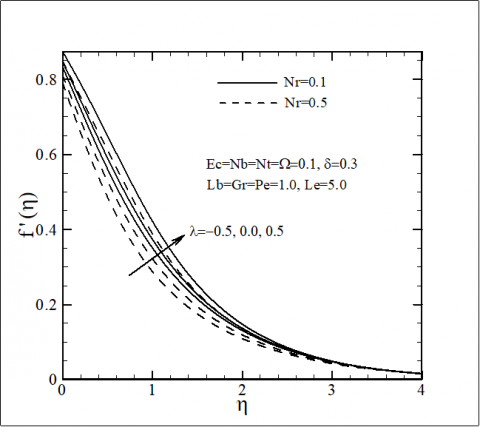Figure 2. The effects of Nr and λ on velocity

## 3.pngFigure 3. The effects of Gr and δ on velocity

## 4.pngFigure 4. The effects of Rb and Ec on velocity

The temperature of nanofluid is high in the region near the sheet. In addition, λ>0 increases the temperature; therefore, it is natural that the maximum temperature happens not at the surface of sheet, but in the neighborhood of it. On the contrary, λ<0 causes the decrease of nanofluid temperature and consequently, the distribution of temperature profiles in λ<0 occurs lower than the distribution of temperature profiles in λ=0. Both of the thermal and flow boundary layer thickness and also the dimensionless temperature increase with increment in Ec. This arises from the fact that increasing in Ec enhances the additional heating due to the viscous dissipation.

## 5.png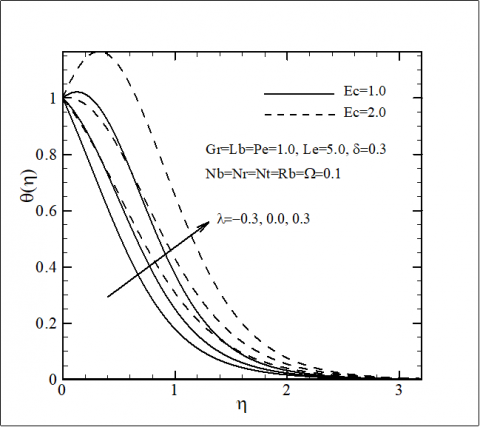Figure 5. The effects of Ec and λ on temperature

Figure 6 illustrates that as δ increase, the temperature slightly enhances. The difference in velocity of the stretching sheet and the neighbor fluid increases as δ enhances, hence, the increase of δ decreases the velocity within boundary layer flow. A reduction in velocity terminates to a decrement in the heat exchange rate and thereupon, causes the enhancement of temperature. As well, it can be seen that an increment in Le enlarges the temperature of nanofluid close to the plate so little and reduces the temperature in the farther distance from the surface. As indicated in Figure 7, thermal boundary layer thickness and temperature, both enhance with the increment of Nb and Nt. The extra heating generated by interaction between the nanoparticles and host fluid as a result of the Brownian movements and thermophoresis influences augments the temperature and the thermal boundary layer thickness.

## 6.pngFigure 6. The effects of Le and δ on temperature

Figure 6 illustrates that as δ increase, the temperature slightly enhances. The difference in velocity of the stretching sheet and the neighbor fluid increases as δ enhances, hence, the increase of δ decreases the velocity within boundary layer flow. A reduction in velocity terminates to a decrement in the heat exchange rate and thereupon, causes the enhancement of temperature. As well, it can be seen that an increment in Le enlarges the temperature of nanofluid close to the plate so little and reduces the temperature in the farther distance from the surface. As indicated in Figure 7, thermal boundary layer thickness and temperature, both enhance with the increment of Nb and Nt. The extra heating generated by interaction between the nanoparticles and host fluid as a result of the Brownian movements and thermophoresis influences augments the temperature and the thermal boundary layer thickness.

## 7.png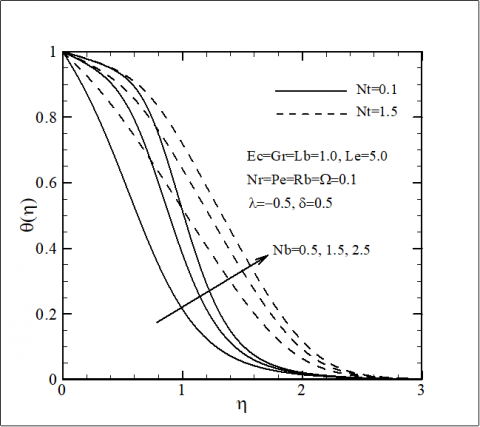Figure 7. The effects of Nt and Nb on temperature

As clearly shown in Figure 8, an increment in Ec boosts boundary layer thickness of the nanoparticles concentration as the nanoparticles concentration reduces. This figure also illustrates that λ<0 and λ>0 make the decrease and increase of nanoparticles concentration near the sheet, respectively. As pointed out earlier, the velocity augments with Ec and λ>0 which causes the decrement of nanoparticles concentration.

## 8.png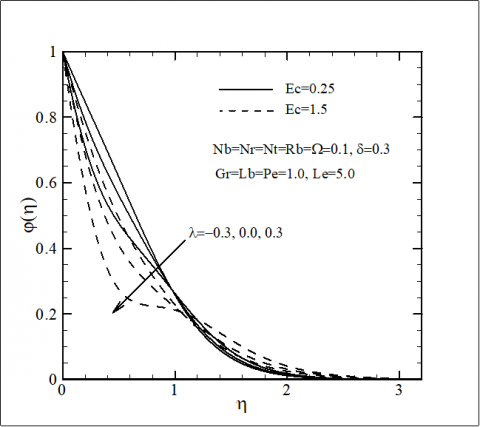Figure 8. The effects of Ec and λ on nanoparticles concentration

Figure 9 illustrates the effects of δ and Le on nanoparticles distribution. As Le enhances, the concentration and the thickness of boundary layer extremely decrease. To justify this observation, it should be pointed out that as Le increases, the convection of nanoparticles increases against the mass diffusion and Brownian motion, hence; both of the concentration and the nanoparticles boundary layer thickness decreases. The nanofluid forced convection decreases as δ increases, therefore it is perspicuous the nanoparticles concentration is increased by increasing δ. Increasing Nt, increases both of the concentration and nanoparticle concentration layer thickness, whereas increment of Nb has the reverse effect of declining on both of them as is shown in Figure 10.

## 9.pngFigure 9. The effects of Le and δ on nanoparticles concentration

## 10.png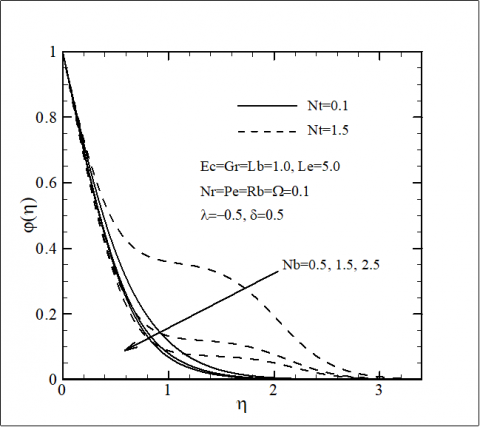Figure 10. The effects of Nb and Nt on nanoparticles concentration

## 11.pngFigure 11. The effects of δ and Pe on density of microorganisms

The effects of different pertinent variables on the non-dimensional density of microorganisms are presented in Figures 11-13. It can be observed increasing Pe, Lb, Gr and Ω decreases both the dimensionless density of motile microorganisms and its thickness, whereas δ makes the increase of the dimensionless density of motile microorganisms within boundary layer.

Figure 12 also indicated that λ<0 and λ>0 decreases and increases the density of microorganisms, respectively. It is noteworthy that λ has not a significant influence on the thickness of boundary layer for motile microorganisms.

## 12.png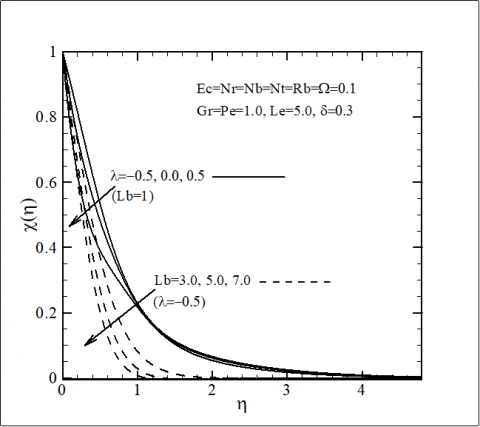Figure 12. The effects of λ and Lb on density of microorganism

## 13.pngFigure 13. The effects of Ω and Gr on density of microorganisms

## 14.pngFigure 14. The effects of λ, Gr and Nr on the reduced skin friction coefficient

The diffusion of microorganisms decrease with enhancing Pe and Lb, hence, it is envisaged that both of density and thickness of boundary layer for microorganisms decreases with an increase in Pe and Lb. Also, it can be stated that decreasing the fluid velocity due to the presence of δ and λ>0 enhances the dimensionless density of microorganisms. In addition, there is a reverse observation when λ<0. The convection caused by the buoyancy force rises with increasing Gr and as a result the density number of the microorganisms declines with an increment in Gr.

An increasing in Gr and Nr decreases and increases Cfr, respectively. Also, it can be observed that the influence of λ on the reduction or enhancement of Cfr increases as Gr amplifies. The variations of Cfr with δ, Ec and Le are pictured in Figure 15. As observed, an increasing in Le, Ec and δ causes the declines of Cfr.

## 15.png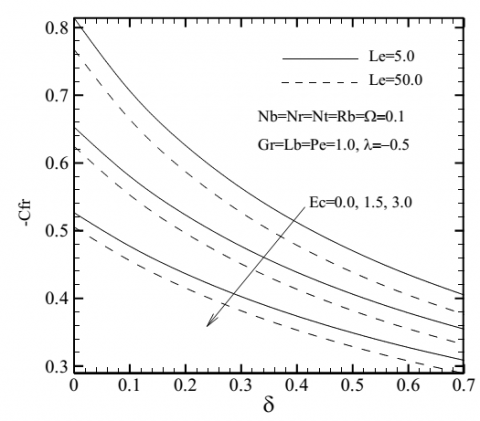Figure 15. The effect of δ, Le and Ec on the reduced skin friction coefficient

## 16.png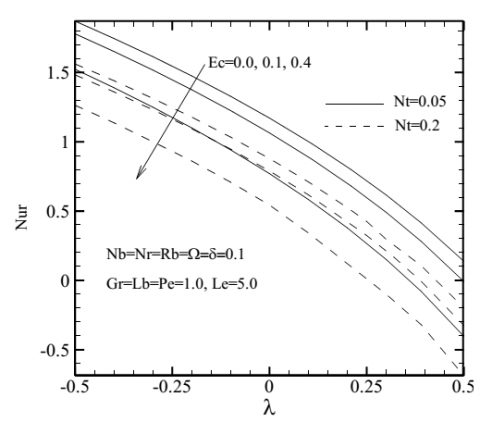Figure 16. The effect of λ, Ec and Nt on the reduced Nusselt number

## 17.png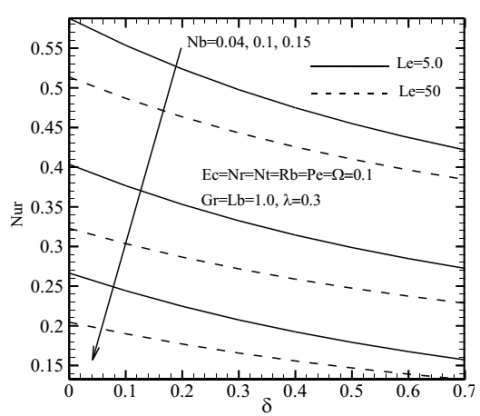Figure 17. The effect of δ, Le and Nb on the reduced Nusselt number

## 18.png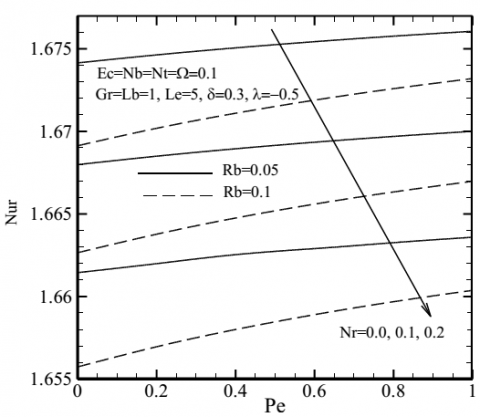Figure 18. The effect of Pe, Nr and Rb on the reduced Nusselt number

## 19.png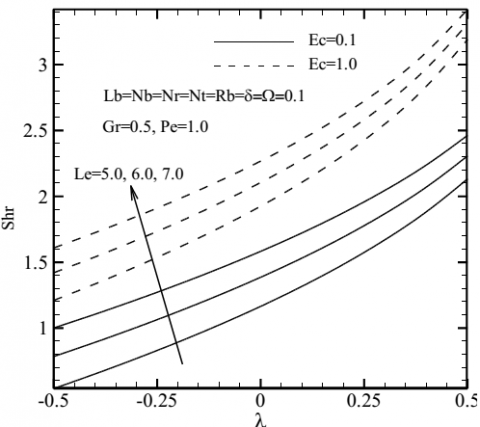Figure 19. The effect of λ, Ec and Le on the reduced Sherwood number

As depicted in Figures 16 and 17, Nur the representative of heat transfer rate, diminishes with increasing Ec, Nt, Nb, δ, Le and λ>0, while increases with λ<0.

As noted in Figures 4-7, the fluid temperature in the boundary layer increases with increment of Ec, Nt, Nb, δ, Le and λ>0 and decreases with λ<0 and as a result Nur decreases with Ec, Nt, Nb, δ, Le and λ>0 and increases with λ<0. Fig. 18 illustrates that the increase of Pe rises the heat transfer rate at the sheet, whereas an increment in Nr and Rb decline reduced Nusselt number Nur. Indeed, this is for the dimensionless temperature enhances because of the decrease of velocity with Rb and Nr.

The variations of reduced Sherwood number Shr with various parameters are illustrated in Figures 19 and 20. It is obvious that Shr increases with an increasing in Ec, Le, Nb and λ>0 and decreases with Nt, δ and λ<0. The influences of the different variables on the reduced density number of the motile microorganisms Nnr are displayed in Figures 21 and 22. As it is shown, Nnr increases with an increasing in Gr, Lb, Pe, Ω and λ>0 while an increasing in δ and λ<0 causes the decrease of Cfr. As mentioned before, the augmentation of Lb and Pe amplifies the convection of motile microorganisms against their diffusion, accordingly, the microorganisms concentration reduces and the concentration gradient rises near the flat plate with increasing Lb and Pe. Also was said that δ and λ<0 reduce the dimensionless velocity and increase the density of motile microorganisms in boundary layer. Consequently, Cfr decreases with an increase in δ and λ<0.

## 20.png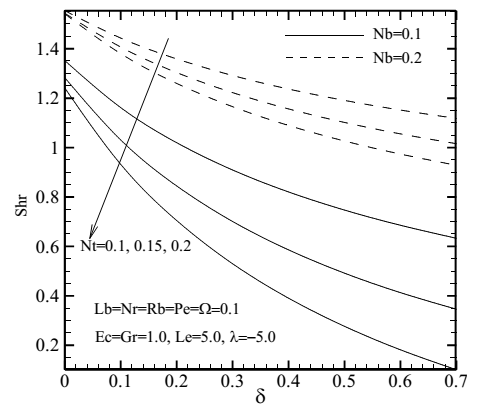Figure 20. The effects of δ, Nb and Nt on the reduced Sherwood number

Increasing the dimensionless velocity in boundary layer due to enhancement Gr diminishes the density of motile microorganisms and as a result increases the motile microorganisms transfer rate from the stretching plate.

## 21.png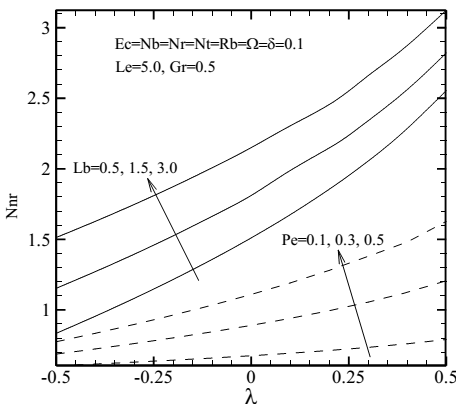Figure 21. The effects of λ, Lb and Pe on the reduced density number of the motile microorganism

## 22.png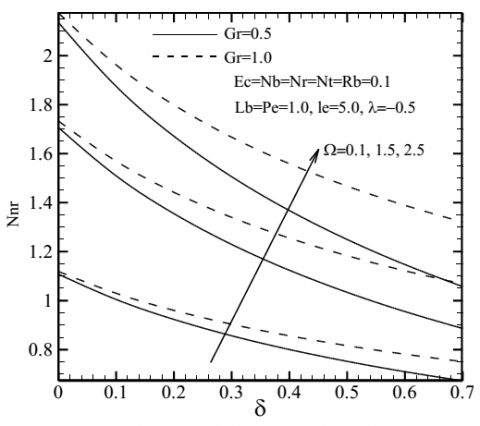Figure 22. The effects of δ, Ω and Gr on the reduced densitynumber of the motile microorganisms

5. Conclusion

Behavior of a nanofluid consisting of gyrotactic microorganisms passing a stretching sheet with considering the impacts of heat absorption/generation and partial slip at the surface is numerically conducted in current investigation. The governing partial differential equations are shifted to a system of the non-linear, coupled and ordinary differential equations using transformations a system of similarity and are solved by the finite difference method with linearization technique of Newton. Numerical results of velocity, temperature, concentration of nanoparticles and density of microorganisms as well as reduced skin friction coefficient Cfr, reduced Nusselt number Nur, reduced Sherwood number Shr and reduced density number of the microorganisms Nnr are represented and discussed in detail. The major findings of this study are outlined below:

1. The dimensionless velocity of nanofluid increases with the increment in Ec, Gr and λ>0 while reduces with δ, Rb, Nr and λ<0. Also, it is found that these parameters have no impact on the thickness of momentum boundary layer.
2. The dimensionless temperature of nanofluid increases as Ec, Nb, Nt, δ and λ>0 increases and decreases in the presence of λ<0. Also, it is seen that as Le increases the temperature increases near the stretching plate so little and reduces in the farther distance from the surface.
3. The nanoparticles concentration increases as δ, Nt and λ<0 increase while decreases with the increase of Nb, Le, Ec and λ>0. An increase in Le and Nt extremely enhance the concentration boundary layer thickness. Additionally, it is observed that the nanoparticles concentration boundary layer thickness slightly boosts with an enhancing in Ec.
4. The dimensionless density of motile microorganisms increases as δ and λ>0 increase while decreases as Pe, Lb, Gr, Ω and λ<0 increase. It is also observed that thickness of boundary layer for motile microorganisms declines with Lb and Ω.
5. As Gr, Le, Ec, δ and λ>0 increase, the reduced skin friction coefficient decreases while augments with the increment of Nr and λ<0.
6. The reduced Nusselt Nur decreases with increasing Ec, Nt, Nb, δ, Le, Rb, Nr and λ>0, while increases with Pe and λ<0.
7. An increasing in Le, Ec, Nb and λ>0 cause the enhancement of the reduced Sherwood Shr while increase in δ, Nt and λ<0 cause the reduction of Shr.

The reduced density of the motile microorganisms Nnr increases as Gr, Ω, Lb, Pe and λ>0 increase and decreases as δ and λ<0 increase.

References

 Hoghoughi G, Izadi M, Oztop HF, Abu-Hamdeh N. (2018). Effect of geometrical parameters on natural convection in a porous undulant-wall enclosure saturated by a nanofluid using Buongiorno's model. Journal of Molecular Liquids 255: 148-159. https://doi.org/10.1016/j.molliq.2018.01.145

 Izadi M, Shahmardan MM, Maghrebi MJ, Behzadmehr A. (2013). Numerical study of developed laminar mixed convection of Al2O3/water nanofluid in an annulus. Chemical Engineering Communications 200(7): 878–894. https://doi.org/10.1080/00986445.2012.723077

 Mehryan SA, Izadi M, Sheremet MA. (2018). Analysis of conjugate natural convection within a porous square enclosure occupied with micropolar nanofluid using local thermal non-equilibrium model. Journal of Molecular Liquids 250: 353–368. http://dx.doi.org/10.1016/j.molliq.2017.11.177

 Izadi M, Mohebbi R, Karimi D, Sheremet MA. (2018). Numerical simulation of natural convection heat transfer inside a┴ shaped cavity filled by a MWCNT-Fe3O4/water hybrid nanofluids using LBM. Chemical Engineering and Processing: Process Intensification 125: 56-66. https://doi.org/10.1016/j.cep.2018.01.004

 Mohebbi R, Izadi M, Chamkha AJ. (2017). Heat source location and natural convection in a C-shaped enclosure saturated by a nanofluid. Physics of Fluids 29(2017): 122009. http://dx.doi.org/10.1063/1.4993866

 Izadi M, Behzadmehr A, Shahmardan MM. (2014). Effects of discrete source-sink arrangements on mixed convection in a square cavity filled by nanofluid. Korean Journal of Chemical Engineering 31(2014): 12-19. http://dx.doi.org/10.1007/s11814-013-0176-7

 Mohebbi R, Rashidi MM, Izadi M, Sidik NAC, Xian HW. (2018). Forced convection of nanofluids in an extended surfaces channel using lattice Boltzmann method. International Journal of Heat and Mass Transfer 117(2018): 1291–1303.

https://doi.org/10.1016/j.ijheatmasstransfer.2017.10.063

 Tadmor Z, Klein I. (1970). Engineering principles of plasticating extrusion. Van Nostrand Reinhold Co.

 Fisher EG. (1976). Extrusion of plastics.

 Altan T, Oh S, Gegel G. (1983). Metal forming fundamentals and applications. American Society for Metals 1983(1983): 353.

 Sakiadis BC. (1961). Boundary‐layer behavior on continuous solid surfaces: I. Boundary‐layer equations for two‐dimensional and axisymmetric flow. AIChE Journal 7(1961): 26–28. https://doi.org/10.1002/aic.690070108

 Le Erickson, Fan LT, Fox VG. (1966). Heat and mass transfer on moving continuous flat plate with suction or injection. Industrial & Engineering Chemistry Fundamentals 5(1966): 19-25. https://doi.org/10.1021/i160017a004

 Crane LJ. (1970). Flow past a stretching plate, Zeitschrift für angewandte Mathematik und Physik ZAMP 21(1970): 645–647.

 Char MI. (1988). Heat transfer of a continuous, stretching surface with suction or blowing. Journal of Mathematical Analysis and Applications 135(2): 568–580. https://doi.org/10.1016/0022-247X(88)90172-2

 Kuznetsov AV, Nield DA. (2010). Natural convective boundary-layer flow of a nanofluid past a vertical plate. International Journal of Thermal Sciences 49(2): 243-247. https://doi.org/10.1016/j.ijthermalsci.2009.07.015

 Khan WA, Pop I. (2010). Boundary-layer flow of a nanofluid past a stretching sheet. International Journal of Heat and Mass Transfer 53(11-12): 2477–2483. https://doi.org/10.1016/j.ijheatmasstransfer.2010.01.032

 Khan WA, Khan ZH, Haq RU. (2015). Flow and heat transfer of ferrofluids over a flat plate with uniform heat flux. The European Physical Journal Plus 130(2015): 86.

 Makinde OD, Aziz A. (2011). Boundary layer flow of a nanofluid past a stretching sheet with a convective boundary condition. International Journal of Thermal Sciences 50(7): 1326–1332. https://doi.org/10.1016/j.ijthermalsci.2011.02.019

 Ibrahim W, Shankar B. (2013). MHD boundary layer flow and heat transfer of a nanofluid past a permeable stretching sheet with velocity, thermal and solutal slip boundary conditions. Computers & Fluids 75: 1-10. https://doi.org/10.1016/j.compfluid.2013.01.014

 Ferdows M, Uddin MJ, Afify AA. (2013). Scaling group transformation for MHD boundary layer free convective heat and mass transfer flow past a convectively heated nonlinear radiating stretching sheet. International Journal of Heat and Mass Transfer 56(1-2): 181-187. https://doi.org/10.1016/j.ijheatmasstransfer.2012.09.020

 Vajravelu K, Prasad KV, Lee J, Lee C, Pop I, van Gorder RA. (2011). Convective heat transfer in the flow of viscous Ag–water and Cu–water nanofluids over a stretching surface. International Journal of Thermal Sciences 50(5): 843–851. https://doi.org/10.1016/j.ijthermalsci.2011.01.008

 Mabood F, Das K. (2016). Melting heat transfer on hydromagnetic flow of a nanofluid over a stretching sheet with radiation and second-order slip. The European Physical Journal Plus 131(1): 3. https://doi.org/10.1140/epjp/i2016-16003-1

 Dehsara M, Dalir N, Nobari MRH. (2014). Numerical analysis of entropy generation in nanofluid flow over a transparent plate in porous medium in presence of solar radiation, viscous dissipation and variable magnetic field. Journal of Mechanical Science and Technology 28(5): 1819–1831.

 Zhu J, Zheng L, Zheng L, Zhang X. (2015). Second-order slip MHD flow and heat transfer of nanofluids with thermal radiation and chemical reaction. Applied Mathematics and Mechanics 36(9): 1131-1146. https://doi.org/10.1007/s10483-015-1977-6

 Seth GS, Mishra MK. (2017). Analysis of transient flow of MHD nanofluid past a non-linear stretching sheet considering Navier’s slip boundary condition. Advanced Powder Technology 28(2): 375–384. https://doi.org/10.1016/j.apt.2016.10.008

 Hayat T, Imtiaz M, Alsaedi A. (2015). Partial slip effects in flow over nonlinear stretching surface. Applied Mathematics and Mechanics 36(11): 1513-1526.

 Serna J. (2016). Heat and mass transfer mechanisms in nanofluids boundary layers. International Journal of Heat and Mass Transfer 92: 173–183. https://doi.org/10.1016/j.ijheatmasstransfer.2015.08.072

 Gorla RSR, Gireesha BJ. (2016). Dual solutions for stagnation-point flow and convective heat transfer of a Williamson nanofluid past a stretching/shrinking sheet. Heat and Mass Transfer 52(6): 1153–1162. https://doi.org/10.1007/s00231-015-1627-y

 Hayat T, Qayyum S, Shehzad SA, Alsaedi A. (2017). Simultaneous effects of heat generation/absorption and thermal radiation in magnetohydrodynamics (MHD) flow of Maxwell nanofluid towards a stretched surface. Results in Physics 7: 562–573. https://doi.org/10.1016/j.rinp.2016.12.009

 Elbashbeshy EM, Emam TG, Abdel-Wahed MS. (20144). An exact solution of boundary layer flow over a moving surface embedded into a nanofluid in the presence of magnetic field and suction/injection. Heat and Mass Transfer 50(1): 57–64.

 Hill NA, Pedley TJ. (2005). Bioconvection. Fluid Dynamics Research 37(1-2): 1–20. https://doi.org/10.1016/j.fluiddyn.2005.03.002

 Avramenko AA, Kuznetsov AV. (2004). Stability of a suspension of gyrotactic microorganisms in superimposed fluid and porous layers. International Communications in Heat and Mass Transfer 31(8): 1057–1066. https://doi.org/10.1016/j.icheatmasstransfer.2004.08.003

 Nield DA, Kuznetsov AV. (2006). The onset of bio-thermal convection in a suspension of gyrotactic microorganisms in a fluid layer: oscillatory convection. International Journal of Thermal Sciences 45(10): 990–997. https://doi.org/10.1016/j.ijthermalsci.2006.01.007

 Alloui Z, Nguyen TH, Bilgen E. (2007). Numerical investigation of thermo-bioconvection in a suspension of gravitactic microorganisms. International Journal of Heat and Mass Transfer 50(7-8): 1435–1441. https://doi.org/10.1016/j.ijheatmasstransfer.2006.09.008

 Kuznetsov AV. (2010). The onset of nanofluid bioconvection in a suspension containing both nanoparticles and gyrotactic microorganisms. International Communications in Heat and Mass Transfer 37(10): 1421–1425. https://doi.org/10.1016/j.icheatmasstransfer.2010.08.015

 Kuznetsov AV. (2011). Nanofluid bioconvection in water-based suspensions containing nanoparticles and oxytactic microorganisms: Oscillatory instability. Nanoscale Research Letters 6(1): 100. https://doi.org/10.1186/1556-276X-6-100

 Kuznetsov AV. (2011). Non-oscillatory and oscillatory nanofluid bio-thermal convection in a horizontal layer of finite depth. European Journal of Mechanics-B/Fluids 30(2): 156–165.

https://doi.org/10.1016/j.euromechflu.2010.10.007

 Aziz A, Khan WA, Pop I. (2012). Free convection boundary layer flow past a horizontal flat plate embedded in porous medium filled by nanofluid containing gyrotactic microorganisms. International Journal of Thermal Sciences 56: 48–57. https://doi.org/10.1016/j.ijthermalsci.2012.01.011

 Mutuku WN, Makinde OD. (2014). Hydromagnetic bioconvection of nanofluid over a permeable vertical plate due to gyrotactic microorganisms. Computers & Fluids 95(22): 88–97. https://doi.org/10.1016/j.compfluid.2014.02.026

 Khan WA, Uddin MJ, Ismail AM. (2013). Free convection of non-Newtonian nanofluids in porous media with gyrotactic microorganisms. Transport in Porous Media 97(2): 241–252.

 Mehryan SA, Kashkooli FM, Soltani M, Raahemifar K. (2016). Fluid flow and heat transfer analysis of a nanofluid containing motile gyrotactic micro-organisms passing a nonlinear stretching vertical sheet in the presence of a non-uniform magnetic field; numerical approach. PloS One 11(6): e0157598. https://doi.org/10.1371/journal.pone.0157598

 Khan WA, Makinde OD, Khan ZH. (2014). MHD boundary layer flow of a nanofluid containing gyrotactic microorganisms past a vertical plate with Navier slip. International Journal of Heat and Mass Transfer 74: 285–291. https://doi.org/10.1016/j.ijheatmasstransfer.2014.03.026

 Hakeem AA, Ganesh NV, Ganga B. (2015). Magnetic field effect on second order slip flow of nanofluid over a stretching/shrinking sheet with thermal radiation effect. Journal of Magnetism and Magnetic Materials 381: 243–257. https://doi.org/10.1016/j.jmmm.2014.12.010

 Sharma R, Ishak A, Pop I. (2016). Stagnation point flow of a micropolar fluid over a stretching/shrinking sheet with second-order velocity slip. Journal of Aerospace Engineering 29(5): 4016025. https://doi.org/10.1061/(ASCE)AS.1943-5525.0000616

 Wang CY. (2003). Flow over a surface with parallel grooves. Physics of Fluids 15(5): 1114–1121. https://doi.org/10.1063/1.1560925

 Sharipov F, Seleznev V. (1998). Data on internal rarefied gas flows. Journal of Physical and Chemical Reference Data 27(3): 657–706. https://doi.org/10.1063/1.556019

 Navier C. (1872). Sur les lois de l’équilibre et du mouvement des corps élastiques, Mem. Acad. R. Sci. Inst. France 6.

 Fang T, Zhang J, Yao S. (2009). Slip MHD viscous flow over a stretching sheet–an exact solution. Communications in Nonlinear Science and Numerical Simulation 14(11): 3731–3737. https://doi.org/10.1016/j.cnsns.2009.02.012

 Nandeppanavar MM, Vajravelu K, Abel MS, Siddalingappa MN. (2012). Second order slip flow and heat transfer over a stretching sheet with non-linear Navier boundary condition. International Journal of Thermal Sciences 58: 143–150. https://doi.org/10.1016/j.ijthermalsci.2012.02.019

 Andersson HI. (2012). Slip flow past a stretching surface. Acta Mechanica 158(1): 121–125. https://doi.org/10.1007/BF01463174

 Noghrehabadi A, Pourrajab R, Ghalambaz M. (2013). Flow and heat transfer of nanofluids over stretching sheet taking into account partial slip and thermal convective boundary conditions. Heat and Mass Transfer 49(9): 1357–1366. https://doi.org/10.1007/s00231-013-1179-y

 Sahoo B, Do Y. (2010). Effects of slip on sheet-driven flow and heat transfer of a third grade fluid past a stretching sheet. International Communications in Heat and Mass Transfer 37(8): 1064–1071. https://doi.org/10.1016/j.icheatmasstransfer.2010.06.018

 Mansur S, Ishak A, Pop I. (2014). Flow and heat transfer of nanofluid past stretching/shrinking sheet with partial slip boundary conditions. Applied Mathematics and Mechanics 35(11): 1401–1410.

 Ariel PD. (2007). Axisymmetric flow due to a stretching sheet with partial slip. Computers & Mathematics with Applications 54(7-8): 1169–1183. https://doi.org/10.1016/j.camwa.2006.12.063

 Aziz A. (2010). Hydrodynamic and thermal slip flow boundary layers over a flat plate with constant heat flux boundary condition. Communications in Nonlinear Science and Numerical Simulation 15(3): 573–580. https://doi.org/10.1016/j.cnsns.2009.04.026

 Noghrehabadi A, Saffarian MR, Pourrajab R, Ghalambaz M. (2013). Entropy analysis for nanofluid flow over a stretching sheet in the presence of heat generation/absorption and partial slip. Journal of Mechanical Science and Technology 27(3): 927–937.

 Azam M, Khan M, Alshomrani AS. (2017). Effects of magnetic field and partial slip on unsteady axisymmetric flow of Carreau nanofluid over a radially stretching surface. Results in Physics 7: 2671–2682. https://doi.org/10.1016/j.rinp.2017.07.025

 Thammanna GT, Gireesha BJ, Mahanthesh B. (2017). Partial slip and Joule heating on magnetohydrodynamic radiated flow of nanoliquid with dissipation and convective condition. Results in Physics 7: 2728–2735. https://doi.org/10.1016/j.rinp.2017.07.056

 Sivakumar N, Prasad PD, Raju CS, Varma SV, Shehzad SA. (2017). Partial slip and dissipation on MHD radiative ferro-fluid over a non-linear permeable convectively heated stretching sheet. Results in Physics 7: 1940–1949. https://doi.org/10.1016/j.rinp.2017.06.016

 Noghrehabadi A, Pourrajab R, Ghalambaz M. (2012). Effect of partial slip boundary condition on the flow and heat transfer of nanofluids past stretching sheet prescribed constant wall temperature. International Journal of Thermal Sciences 54: 253–261. https://doi.org/10.1016/j.ijthermalsci.2011.11.017